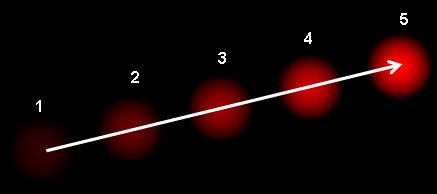# 光流¶

### 光流¶I(x,y,t) = I(x+dx, y+dy, t+dt)

f_x u + f_y v + f_t = 0 \;

u = \frac{dx}{dt} \; ; \; v = \frac{dy}{dt}

\begin{bmatrix} u \\ v \end{bmatrix} = \begin{bmatrix} \sum_{i}{f_{x_i}}^2 & \sum_{i}{f_{x_i} f_{y_i} } \\ \sum_{i}{f_{x_i} f_{y_i}} & \sum_{i}{f_{y_i}}^2 \end{bmatrix}^{-1} \begin{bmatrix} - \sum_{i}{f_{x_i} f_{t_i}} \\ - \sum_{i}{f_{y_i} f_{t_i}} \end{bmatrix}

import numpy as np
import cv2 as cv
import argparse
parser = argparse.ArgumentParser(description='This sample demonstrates Lucas-Kanade Optical Flow calculation. \
https://www.bogotobogo.com/python/OpenCV_Python/images/mean_shift_tracking/slow_traffic_small.mp4')
parser.add_argument('image', type=str, help='path to image file')
args = parser.parse_args()
cap = cv.VideoCapture(args.image)
# 用于ShiTomasi拐点检测的参数
feature_params = dict( maxCorners = 100,
qualityLevel = 0.3,
minDistance = 7,
blockSize = 7 )
lk_params = dict( winSize  = (15,15),
maxLevel = 2,
criteria = (cv.TERM_CRITERIA_EPS | cv.TERM_CRITERIA_COUNT, 10, 0.03))
# 创建一些随机的颜色
color = np.random.randint(0,255,(100,3))
# 拍摄第一帧并在其中找到拐角
old_gray = cv.cvtColor(old_frame, cv.COLOR_BGR2GRAY)
p0 = cv.goodFeaturesToTrack(old_gray, mask = None, **feature_params)
# 创建用于作图的掩码图像
while(1):
frame_gray = cv.cvtColor(frame, cv.COLOR_BGR2GRAY)
# 计算光流
p1, st, err = cv.calcOpticalFlowPyrLK(old_gray, frame_gray, p0, None, **lk_params)
# 选择良好点
good_new = p1[st==1]
good_old = p0[st==1]
# 绘制跟踪
for i,(new,old) in enumerate(zip(good_new, good_old)):
a,b = new.ravel()
c,d = old.ravel()
frame = cv.circle(frame,(a,b),5,color[i].tolist(),-1)
cv.imshow('frame',img)
k = cv.waitKey(30) & 0xff
if k == 27:
break
# 现在更新之前的帧和点
old_gray = frame_gray.copy()
p0 = good_new.reshape(-1,1,2)


（此代码不会检查下一个关键点的正确性。因此，即使任何特征点在图像中消失了，光流也有可能找到下一个看起来可能与它接近的下一个点。因此，对于稳健的跟踪，实际上 应该以特定的时间间隔检测点。OpenCV样本附带了这样一个样本，该样本每5帧发现一次特征点，并且还对光流点进行了后向检查，以仅选择良好的流点。请参阅代码 samples/python/lk_track.py）。### OpenCV中的密集光流¶

import numpy as np
import cv2 as cv
cap = cv.VideoCapture(cv.samples.findFile("vtest.avi"))
prvs = cv.cvtColor(frame1,cv.COLOR_BGR2GRAY)
hsv = np.zeros_like(frame1)
hsv[...,1] = 255
while(1):
next = cv.cvtColor(frame2,cv.COLOR_BGR2GRAY)
flow = cv.calcOpticalFlowFarneback(prvs,next, None, 0.5, 3, 15, 3, 5, 1.2, 0)
mag, ang = cv.cartToPolar(flow[...,0], flow[...,1])
hsv[...,0] = ang*180/np.pi/2
hsv[...,2] = cv.normalize(mag,None,0,255,cv.NORM_MINMAX)
bgr = cv.cvtColor(hsv,cv.COLOR_HSV2BGR)
cv.imshow('frame2',bgr)
k = cv.waitKey(30) & 0xff
if k == 27:
break
elif k == ord('s'):
cv.imwrite('opticalfb.png',frame2)
cv.imwrite('opticalhsv.png',bgr)
prvs = next